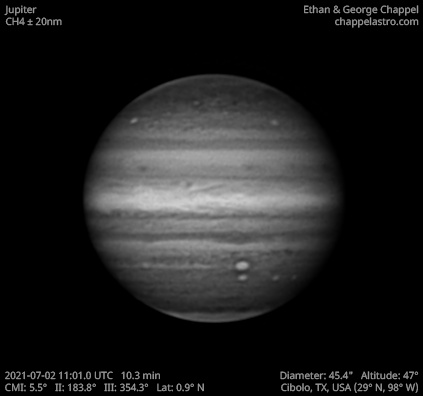# Jupiter 2021-07-02 11:01 UTC

CM1: 5.50°

CM2: 183.80°

CM3: 354.30°

CLat: +0.80°

Description

A beautiful night with excellent seeing July 2, 2021. We were prepared this time and took out the EdgeHD 14 to take high resolution images of Jupiter.

The North Equatorial Belt, or NEB for short, is the most prominent belt in the northern hemisphere of Jupiter. It typically spans from 9 to 17 degrees north of the equator, but the northern edge will occasionally push into the neighboring North Tropical Zone until it reaches 21 degrees north. When this happens, the belt is in an expanded state and usually stays that way for about one Earth year.

When the belt expands northward, two types of storms will emerge from the turbulence: anticyclonic white spots and cyclonic brown barges. Both types of storms will disappear once the NEB recedes southward. One notable exception is known as White Spot Z, which has persisted in the region since 1997.

The NEB is currently receding after an expansion event in 2020. This time, the new northern edge appears to be at 12 degrees north. Northward expansions following these unusually far receding events are typically much more vigorous than typical expansion events, so we may be in for an exciting show anytime from 2023-2025 if this follows the same pattern.

Equipment

ZWO ASI290MM

Celestron EdgeHD 14

Logs
```FireCapture v2.6  Settings
------------------------------------
Observer=Ethan Chappel
Location=Cibolo
Scope=Celestron C14 EdgeHD
Camera=ZWO ASI290MM
Filter=CH4
Profile=Jupiter
Diameter=45.38"
Magnitude=-2.66
CMI=7.2° CMII=185.5° CMIII=356.1°  (during mid of capture)
FocalLength=3000mm (F/8)
Resolution=0.20"
Filename=2021-07-02-1104_6-EC-CH4-Jup.ser
Date=2021_07_02
Start=11_03_07.529
Mid=11_04_37.548
End=11_06_07.567
Start(UT)=11_03_07.529
Mid(UT)=11_04_37.548
End(UT)=11_06_07.567
Duration=180.038s
Date_format=yyyy_MM_dd
Time_format=HH_mm_ss
LT=UT -6h
Frames captured=2572
File type=SER
Binning=no
ROI=464x336
ROI(Offset)=504x40
FPS (avg.)=14
Shutter=70.00ms
Gain=453 (75%)
SoftwareGain=10 (off)
FPS=100 (off)
USBTraffic=50 (off)
AutoHisto=75 (off)
AutoGain=off
AutoExposure=off
Gamma=50 (off)
HighSpeed=off
Brightness=1 (off)
Histogramm(min)=0
Histogramm(max)=171
Histogramm=67%
Noise(avg.deviation)=7.33
Limit=180 Seconds
Sensor temperature=32.6°C
Focuser position=1460
FireCapture v2.6  Settings
------------------------------------
Observer=Ethan Chappel
Location=Cibolo
Scope=Celestron C14 EdgeHD
Camera=ZWO ASI290MM
Filter=CH4
Profile=Jupiter
Diameter=45.38"
Magnitude=-2.66
CMI=5.1° CMII=183.4° CMIII=353.9°  (during mid of capture)
FocalLength=3000mm (F/8)
Resolution=0.20"
Filename=2021-07-02-1101_1-EC-CH4-Jup.ser
Date=2021_07_02
Start=10_59_36.453
Mid=11_01_06.483
End=11_02_36.514
Start(UT)=10_59_36.453
Mid(UT)=11_01_06.483
End(UT)=11_02_36.514
Duration=180.061s
Date_format=yyyy_MM_dd
Time_format=HH_mm_ss
LT=UT -6h
Frames captured=2573
File type=SER
Binning=no
ROI=464x336
ROI(Offset)=504x40
FPS (avg.)=14
Shutter=70.00ms
Gain=453 (75%)
SoftwareGain=10 (off)
FPS=100 (off)
USBTraffic=50 (off)
AutoHisto=75 (off)
AutoGain=off
AutoExposure=off
Gamma=50 (off)
HighSpeed=off
Brightness=1 (off)
Histogramm(min)=0
Histogramm(max)=176
Histogramm=69%
Noise(avg.deviation)=7.33
Limit=180 Seconds
Sensor temperature=32.6°C
Focuser position=1460
FireCapture v2.6  Settings
------------------------------------
Observer=Ethan Chappel
Location=Cibolo
Scope=Celestron C14 EdgeHD
Camera=ZWO ASI290MM
Filter=CH4
Profile=Jupiter
Diameter=45.38"
Magnitude=-2.66
CMI=2.8° CMII=181.2° CMIII=351.7°  (during mid of capture)
FocalLength=2950mm (F/8)
Resolution=0.20"
Filename=2021-07-02-1057_3-EC-CH4-Jup.ser
Date=2021_07_02
Start=10_55_52.567
Mid=10_57_22.572
End=10_58_52.577
Start(UT)=10_55_52.567
Mid(UT)=10_57_22.572
End(UT)=10_58_52.577
Duration=180.010s
Date_format=yyyy_MM_dd
Time_format=HH_mm_ss
LT=UT -6h
Frames captured=2572
File type=SER
Binning=no
ROI=464x336
ROI(Offset)=504x40
FPS (avg.)=14
Shutter=70.00ms
Gain=453 (75%)
SoftwareGain=10 (off)
FPS=100 (off)
USBTraffic=50 (off)
AutoHisto=75 (off)
AutoGain=off
AutoExposure=off
Gamma=50 (off)
HighSpeed=off
Brightness=1 (off)
Histogramm(min)=0
Histogramm(max)=160
Histogramm=62%
Noise(avg.deviation)=7.22
Limit=180 Seconds
Sensor temperature=32.8°C
Focuser position=1460
```